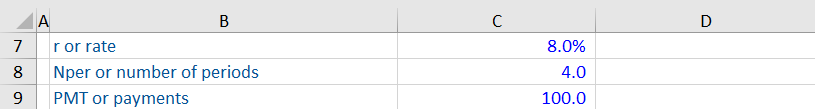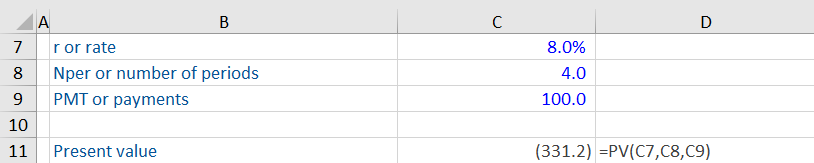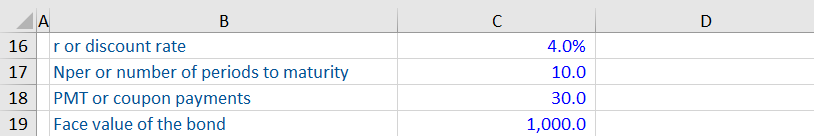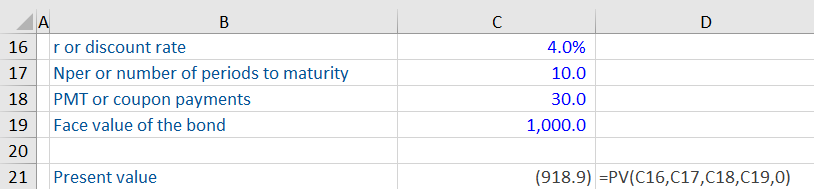## What is the PV Function?

The PV function is a financial function in Excel that calculates the present value of a future stream of cash flows based on a constant interest rate.

The present value is one of the most important concepts in finance as part of the time value of money principle. It helps in comparing different investment alternatives and choosing the most profitable ones. The PV function can either be used with periodic cash inflows/outflows or a one-time payment/income.

## Key Learning Points

• The PV function a financial function in Excel used for calculating the present value of future payments/income based on a constant interest rate
• While the present value can be calculated without the PV function, use of the function greatly increases efficiency
• The present value is a key concept in finance under the time value of money principle. One of the uses of present value is to choose the most profitable investment among different alternatives
• It is important to be consistent with units used to specify rate and nper

## PV Formula

The syntax for the PV function is shown as:

PV (rate, nper, pmt, [fv], type)

The syntax includes the following arguments:

• Rate represents the interest rate. For example, if someone invests in a bond paying an interest rate of 2%, this will be entered as the ‘rate’.
• Nper represents the number of payment periods for which the present value is being calculated. For a bond that matures in 4 years with semi-annual payments, the ‘nper’ will be 8 (4 years x 2 payments every year).
• Pmt represents the amount of payment in each period. For a bond that pays an interest of \$250 per annum, this amount will be included as ‘pmt’.

### Optional Arguments:

• [FV] represents the future value or the balance at the end of the payment periods. For example, you want to know the amount to be invested now to get 100 in 10 years at a rate of 4%. Here, 100 is the future value.
• Type represents when the payment is due. It can either be 0 (payment at the end of the period) or 1 (payment at the beginning of the period).

## Example 1:

What is the present value of an investment that pays 100 every year at 8% for 4 years?Using the PV function, we get the present value of this investment as 331.2. This value is shown as a negative number as it implies a cash outflow, i.e., we need to invest this amount now to get a payment of 100 every year for 4 years.While entering the information, make sure to include each argument individually. After you type =PV in the formula cell, you need to go to C7, then C8, then C9 and so on. Unlike the sum function, you cannot drag all the cells at one go.

## Example 2:

We have been asked to calculate the present value of a 10-year bond paying a coupon of 30.0 per annum. The face value of this bond is 1,000.0, and the interest rate is 4%. The coupon payment is made at the end of each year.We enter the arguments required for the PV function as follows:Notice that we have also entered optional arguments in this example. Here, the face value of the bond represents its future value. And as the payment is made at the end of each year, we’ve entered type as 0.

The present value of this bond is 918.9.

### Important Points about the PV Function:

• The annual interest rate should be converted into a periodic interest rate. For example, if it is a semi-annual bond paying an interest of 3% per annum, the interest rate should be 1.5% (3%/2 payments every year)
• The units used for nper and rate should be consistent. Continuing with the previous example, if it’s a two-year bond, the nper should be 4 (2 years x 2 payments per year).
• If non-numeric arguments are entered, the PV function can give a #Value! error